## Example Questions

### Example Question #1 : Variables

Factor the following variable

(x2 + 18x + 72)

(x – 6) (x – 12)

(x – 6) (x + 12)

(x + 6) (x + 12)

(x + 18) (x + 72)

(x + 6) (x – 12)

(x + 6) (x + 12)

Explanation:

You need to find two numbers that multiply to give 72 and add up to give 18

easiest way: write the multiples of 72:

1, 72

2, 36

3, 24

4, 18

6, 12: these add up to 18

(x + 6)(x + 12)

### Example Question #2 : Variables

Factor 9x2 + 12x + 4.

(3x – 2)(3x – 2)

(3x + 2)(3x + 2)

(9x + 4)(9x + 4)

(3x + 2)(3x – 2)

(9x + 4)(9x – 4)

(3x + 2)(3x + 2)

Explanation:

Nothing common cancels at the beginning. To factor this, we need to find two numbers that multiply to 9 * 4 = 36 and sum to 12. 6 and 6 work.

So 9x2 + 12x + 4 = 9x2 + 6x + 6x + 4

Let's look at the first two terms and last two terms separately to begin with. 9x2 + 6x can be simplified to 3x(3x + 2) and 6x + 4 can be simplified into 2(3x + 2). Putting these together gets us

9x2 + 12x + 4

= 9x2 + 6x + 6x + 4

= 3x(3x + 2) + 2(3x + 2)

= (3x + 2)(3x + 2)

This is as far as we can factor.

### Example Question #2 : Algebra

If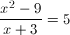, and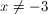, what is the value of?

–6

–8

6

0

8

8

Explanation:

The numerator on the left can be factored so the expression becomes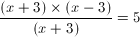, which can be simplified to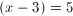Then you can solve forby adding 3 to both sides of the equation, so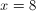### Example Question #2 : Factoring

Solve for x: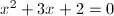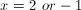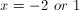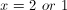Explanation:

First, factor.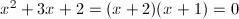Set each factor equal to 0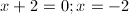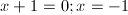Therefore,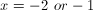### Example Question #1 : Factoring

When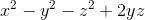is factored, it can be written in the form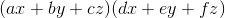, where,,,,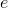, andare all integer constants, and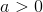.

What is the value of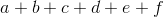?Explanation:

Let's try to factor x2 – y2 – z2 + 2yz.

Notice that the last three terms are very close to y2 + z2 – 2yz, which, if we rearranged them, would become y2 – 2yz+ z2. We could factor y2 – 2yz+ z2 as (y – z)2, using the general rule that p2 – 2pq + q2 = (p – q)2 .

So we want to rearrange the last three terms. Let's group them together first.

x2 + (–y2 – z2 + 2yz)

If we were to factor out a –1 from the last three terms, we would have the following:

x2 – (y2 + z2 – 2yz)

Now we can replace y2 + z2 – 2yz with (y – z)2.

x2 – (y – z)2

This expression is actually a differences of squares. In general, we can factor p2 – q2 as (p – q)(p + q). In this case, we can substitute x for p and (y – z) for q.

x2 – (y – z)= (x – (y – z))(x  + (y – z))

Now, let's distribute the negative one in the trinomial x – (y – z)

(x – (y – z))(x  + (y – z))

(x – y + z)(x + y – z)

The problem said that factoring x2 – y2 – z2 + 2yz would result in two polynomials in the form (ax + by + cz)(dx + ey + fz), where a, b, c, d, e, and f were all integers, and a > 0.

(x – y + z)(x + y – z) fits this form. This means that a = 1, b = –1, c = 1, d = 1, e = 1, and f = –1. The sum of all of these is 2.

### Example Question #1 : Factoring

Factor and simplify: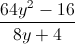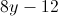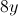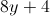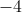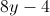Explanation: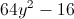is a difference of squares.

The difference of squares formula is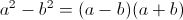.

Therefore,=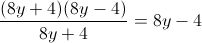.

### Example Question #2 : Factoring

Factor: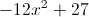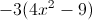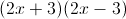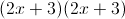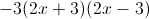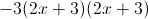Explanation:

We can first factor out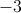:This factors further because there is a difference of squares:### Example Question #1 : How To Factor A Variable

A group of scientists form a global collective of temperature and climate data. U.S. temperature measurements are in Fahrenheit and must be converted to Celsius. If the average spring temperature for New York was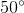Fahrenheit, what is the temperature value in Celsius?

The Fahrenheit to Celsius conversion equation is as follows: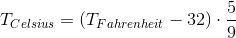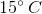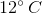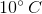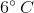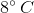Explanation:

You can solve this problem by substituting infor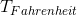and solving for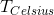: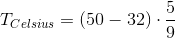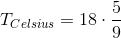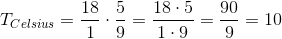That means thatFahrenheit is the same as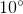Celsius.

### Example Question #1 : How To Factor A Variable

Factor to the simplest form: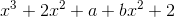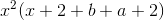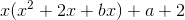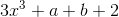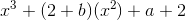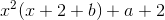Explanation:

Group all the terms with thevariable.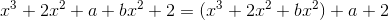Pull out anterm from parentheses.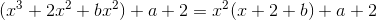There are no more common factors.

The correct answer is:### Example Question #1 : How To Factor A Variable

A semi truck unloaded weighs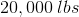. When the trailer compartment is loaded to half capacity with soda, the semi weighs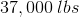. What will the truck weigh when the compartment is loaded tocapacity with the same kind of soda?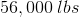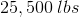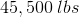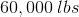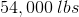Explanation:

This word problem can be broken down into a basic algebraic equation:

A half-loaded semi weighs 37,000 lbs. Subtracting the weight of the truck, we can determine that a half load weighs 17,000 lbs: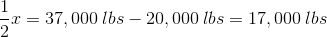From that, we can determine that a full load = 34,000 lbs: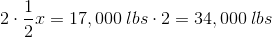Knowing the weight of a full load, we can calculate the weight of a 3/4 load: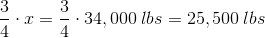Add the weight of the truck to get the total weight: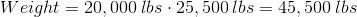### All SAT Math Resources